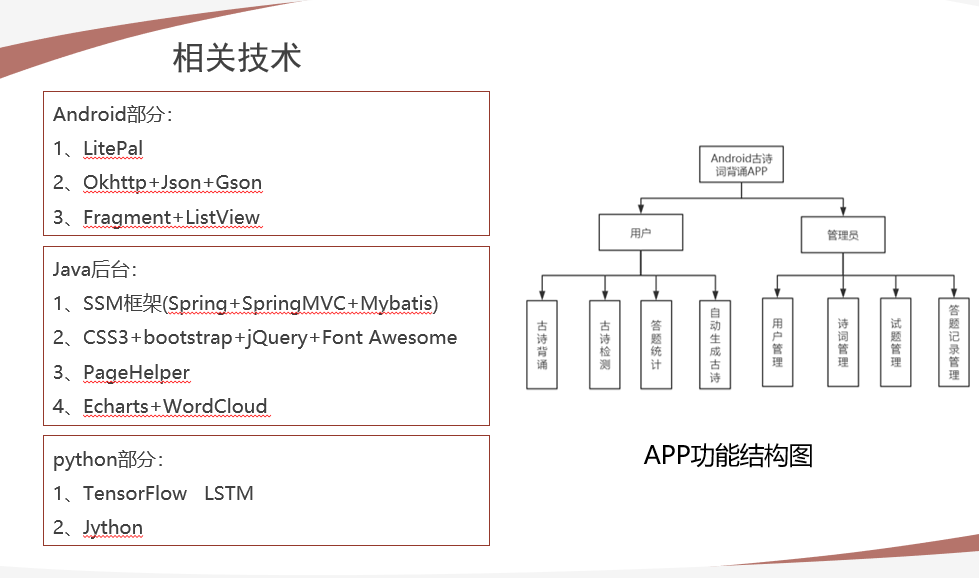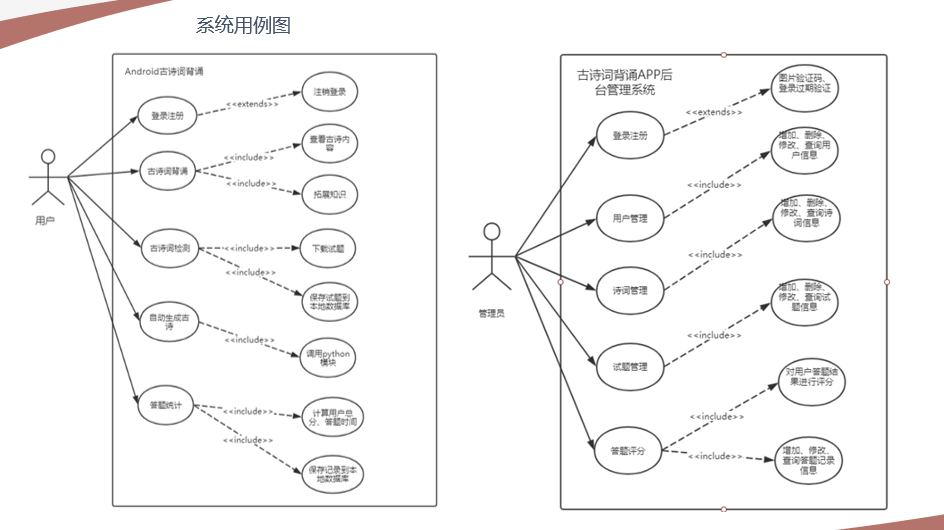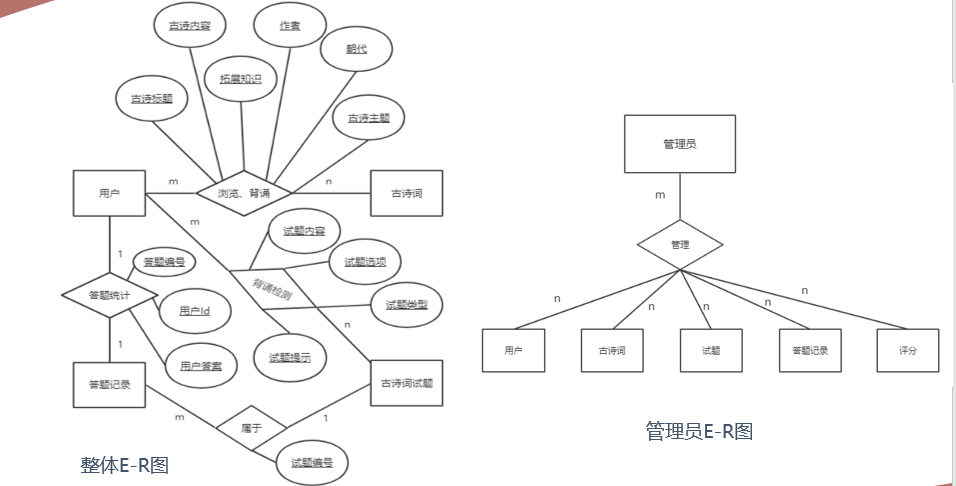• 于web的中小学生古诗词网的设计与实现是把古诗词放在网络中供用户来进行学习，是通过ASP.NET技术平台，古诗词存储到SQL Server数据库中。系统分为了前台学习用户和后台古诗词管理员两种用户类型。前台学习用户主要的...
• 基于“深度学习”的小学语文古诗教学策略探究.pdf
• 数据来源[郑州大学全唐诗库]... 这是因为很多诗歌有多位作者，因此在每句话的后面都注解了作者的名字。但是对于机器学习，或者对于机器来说，无法分辨这些到底是作者名字，还是正式的诗句
• 爬取目标网站的文本，如古诗的内容，作者，朝代，并且保存到本地中。  3、爬虫程序 # -*- coding:utf-8 -*- #爬取古诗网站 import requests import re #下载数据 def write_data(data): with open('诗词.txt','a...
• 指向深度学习古诗教学“四意识”——以部编版小学语文四年级上册第三单元《古诗三首》为例.pdf
• 使用tensorflow深度学习，训练机器写古诗词。唐诗生成
• 深度学习背景下古诗词教学的思考——一首诗远不止是一首诗.pdf
• 深度学习,让学生爱上古诗词——基于三年级的古诗词深度学习策略.pdf
• 小学生课前三分钟古诗风唐李峤PPT学习教案.pptx
• 我们这里以中国古诗为例，我们将要构造一个RNN 模型，并用它来自动生成古诗。 准备工作： 我们拿到的是一个txt文件，里面包含了一些古诗。 我们要做的是一下几点： 分割出古诗主体部分，去掉所有的标题或是作者...
这是一个RNN的典型应用，也是RNN最能解决的一个应用场景。 我们这里以中国古诗为例，我们将要构造一个RNN 模型，并用它来自动生成古诗。
准备工作：
我们拿到的是一个txt文件，里面包含了一些古诗。 我们要做的是一下几点：
分割出古诗主体部分，去掉所有的标题或是作者信息。找出这个文件中的所有汉字，另外再加入三个英文字母： C（逗号）、D（句号）、E（结尾标志）。生成两个字典，分别是word2vec 以及 vec2word。 这两个字典是很经典的在处理NLP时必要的东西。生成features 以及 labels
注意事项：
txt文件编码改为UTF-8.对于以上的第四点， 对于一句古诗, 例如： #####床前明月光C疑似地上霜D举头望明月C低头思故乡。E 我们的 feature应该是 #####床前明月光C疑似地上霜D举头望明月C低头思故乡。 而label则是： #####前明月光C疑似地上霜D举头望明月C低头思故乡。E 即feature 和 label间隔为1
RNN
我们将会使用LSTM 来训练。
数据清洗
我们使用的文件本身并不是很友好，经过考虑之后，决定先进行数据处理，从文件中提取出所有的五言律诗来训练。 代码如下： 这里我们先读取文件， 将文件中的所有标点符号去掉，保留逗号、句号以及手动加上末尾，将所有的诗句保存在一个list里面。
with open('./poems.txt','r',encoding='UTF-8') as f:
content = []
for line in f:
try:
_ , sentence = line.split(":")
except Exception as Exc:
continue
sentence = sentence.replace(' ', '').replace('\n', '').replace('__','')
if set('()（））_《》[]') & set(sentence):
continue
if begin in sentence or end in sentence:
continue
if len(sentence) < 5 or len(sentence) > 79:
continue
sentence = sentence.replace('，', 'C').replace('。','D')
sentence += end
content.append(sentence)

print(type(content))
print(len(content))
poem_num = len(content)
####
with open('./content.txt', 'a', encoding='utf-8') as con:
for poem in content:
con.write(poem)
con.write('\n')

接着，我们提取出所有可能的诗歌长度。
length_dict = {}
length_list = []
for poem in content:
l = len(poem)
if l not in length_dict:
length_dict[l] = 1
if l not in length_list:
length_list.append(l)
length_list.sort()
print(length_list)

然后，按照诗歌长度对整个文件进行分类， 并另存为以诗歌长度为名字的txt文件里面。
for l in length_dict:
for poem in content:
if len(poem) == l:
file_path = './' + str(l) + '.txt'
with open(file_path, 'a', encoding = 'utf-8') as f:
f.write(poem)
f.write('\n')
content.remove(poem)

这样数据就已经清理好啦。 五言律诗的长度为49， 我这里直接把这个文件手动重命名为poem.txt。
Features and Labels
我们需要将文字转成数字张量才能进行训练。虽然我们做了数据处理，但是五言律诗依旧有两千多首，我们决定先拿其中的100首作为练习。
#load packages:
import numpy as np
import keras
from tqdm import tqdm

batch = 100

读取文件


with open('./poem.txt','r',encoding='UTF-8') as f:
content = []
for line in f:
content.append(line)

#print(type(content))
#print(len(content))
poem_num = len(content)
content_temp = content[0:batch - 1]

#print(content)
#print(len(content))

#print('/n')
#print(content[-1])

这里我们要构造两个经典的dict, 分别是word2vec 和vec2word。 首先，我们找出这五百首诗中一共有多少的汉字。
word_dict = {}
for c in content_temp:
for w in c:
if w not in word_dict:
word_dict[w] = 1

#print(len(word_dict))

#### collect all of words
word_list = []
for w in word_dict:
word_list.append(w)
#print(len(word_list))

max_length = len(word_list)


接着构造字典
word2vec = {}
num2word = {}
word2num = {}

def vec_generator(length,k):
vec = np.zeros(length)
vec[k] = 1
return vec

#### creating mapping between words and nums
for i,j in enumerate(word_list):
word2vec[j] = vec_generator(len(word_list), i)
num2word[i] = [j]
word2num[j] = [i]


有了这个字典之后，便可以开始构造features 和 lables
def sentence2feature(sentence):
feature = []
for s in sentence[:-1]:
feature.append(word2vec[s])

feature = np.array(feature)
return np.expand_dims(feature, axis = 0)

def sentence2label(sentence):
labels = []
for s in sentence[1:]:
labels.append(word2vec[s])

labels = np.array(labels)

return np.expand_dims(labels , axis = 0)

features = sentence2feature(content_temp)
labels = sentence2label(content_temp)
for i in tqdm(range(batch-1)):
poem = content_temp[i]
word_num = sentence2feature(poem)
label_num = sentence2label(poem)
features = np.append(features, word_num, axis = 0)
labels = np.append(labels, label_num, axis = 0)

print(features.shape)
print(labels.shape)

输出如下：
(100, 48, 1)
(100, 48, 1335)

意思是，我们从文件中提取了100首诗， 这些诗歌中一共包含了1332个汉字以及逗号、句号和END。 我们的feature是由整首诗组成的，我们期望的是，feature中的最后一个元素进入model之后会输出END。
RNN
这里我们就使用简单的RNN来训练
model = keras.Sequential()
model.add(keras.layers.LSTM(units = 4096, input_dim = 1, input_length = None,
return_sequences = True))
#model.add(keras.layers.LSTM(units = 4096, return_sequences = True))
opt = keras.optimizers.rmsprop(lr=0.001, decay=1e-6)
model.compile(optimizer = opt,
loss = 'categorical_crossentropy',
metrics = ['accuracy'])
model.summary()

其中，return_sequences = True， 因为我们需要在每一个cell上面做输出。
当模型训练完成之后，我们便可以利用这个模型进行古诗词的自动输出。这里我们需要自己选一个汉字作为开头。因为在RNN 模型中，我们使用了dynamic的功能。我们并没有要求输入的数据需要有48个时间步长，因此，当选定一个汉字，例如“明” 之后，我们利用word2num函数将其转换成数字，并且利用numpy自导的功能转换成一个三维的矩阵。这时候，时间步长为1，我们将会得到一个输出，y。 将y转换成数字之后，我们将之前的输入与y进行连接，并作为新的输入。 以此类推。
init = '明'
poem = init
init_num = word2num[init]
feature = np.array([[init_num]])
while True:
predict = model.predict(feature)
predict_num = np.argmax(predict[0, -1, :])
if num2word[predict_num] == 'E':
break
else:
poem += num2word[predict_num][-1]
predict_feature = np.array([[predict_num]])
predict_feature = np.expand_dims(predict_feature, axis = 0)
feature = np.append(feature,predict_feature, axis = 1 )

if len(poem) == 49:
break

poem = poem.replace('C','，').replace('D', '。').replace('E','')
print(poem, '\n')
for i in range(4):
print(poem[i * 12: (i+1) * 12])

结果如下： 明角出塞门，前瞻即胡地。 三军尽回首，皆洒望乡泪。 转念关山长，行看风景异。 由来征戍客，各负轻生义。
####################################################################### update:
更新数据清洗方式：
with open('./poems.txt', 'r', encoding='UTF-8') as f:
with open("training_set.txt","w+") as t:
for line in f:
idx = line.index(":")
if len(line[idx: -1]) == 49 and line[idx+1: -1].index("，") == 5 and "□" not in line:
t.write(line[idx+1: -1].replace("，", "C").replace("。", "D") + "E" + "\n")

之前的处理方式并不是很好，因为诗词中包含了一些乱码，以及括号引用。 我们现在用以下判别： 1、 找出冒号所在的位子，冒号之后的长度应该为49。 2、 因为我们要找五言绝句， 那么这首诗里面的每一句话长度应该为5。 因此，从冒号开始，到第一个逗号的长度应该为5。 3、 去除乱码。
展开全文人工智能 TensorFlow Keras
• 内置海量中文数据，可以自主生成古诗词，有助于教书教学
• 基于Android系统的古诗词背诵APP主要是一个在面向所有想快速学习古诗词的用户以快捷方便、操作简单、实时反馈、古风主题为特点的一个APP，该APP的主要任务是方便人们在线背诵记忆自己感兴趣的古诗词，或者进行背诵...
项目名称：古诗词学习APP系统相关图示：后端管理系统开发，参考SSM框架视频：

https://www.bilibili.com/video/av35988777?from=search&seid=1394276140344891694

项目上传至我的git： https://github.com/Rookie1996/PoetryRecite
展开全文Android web开发 java...